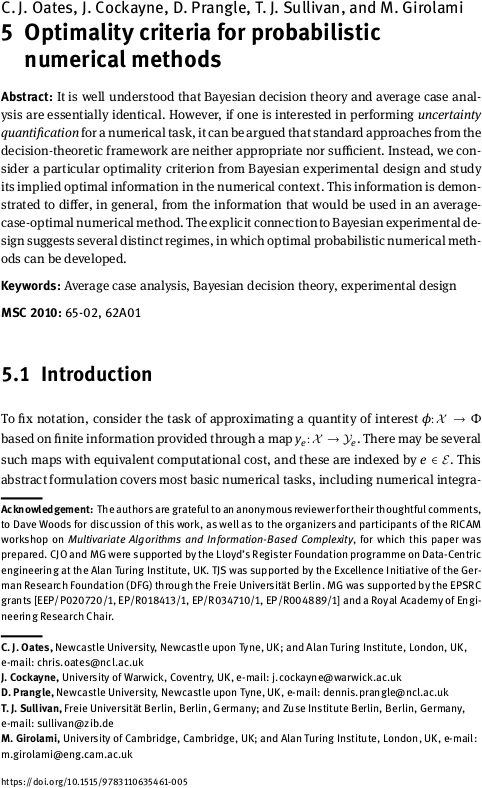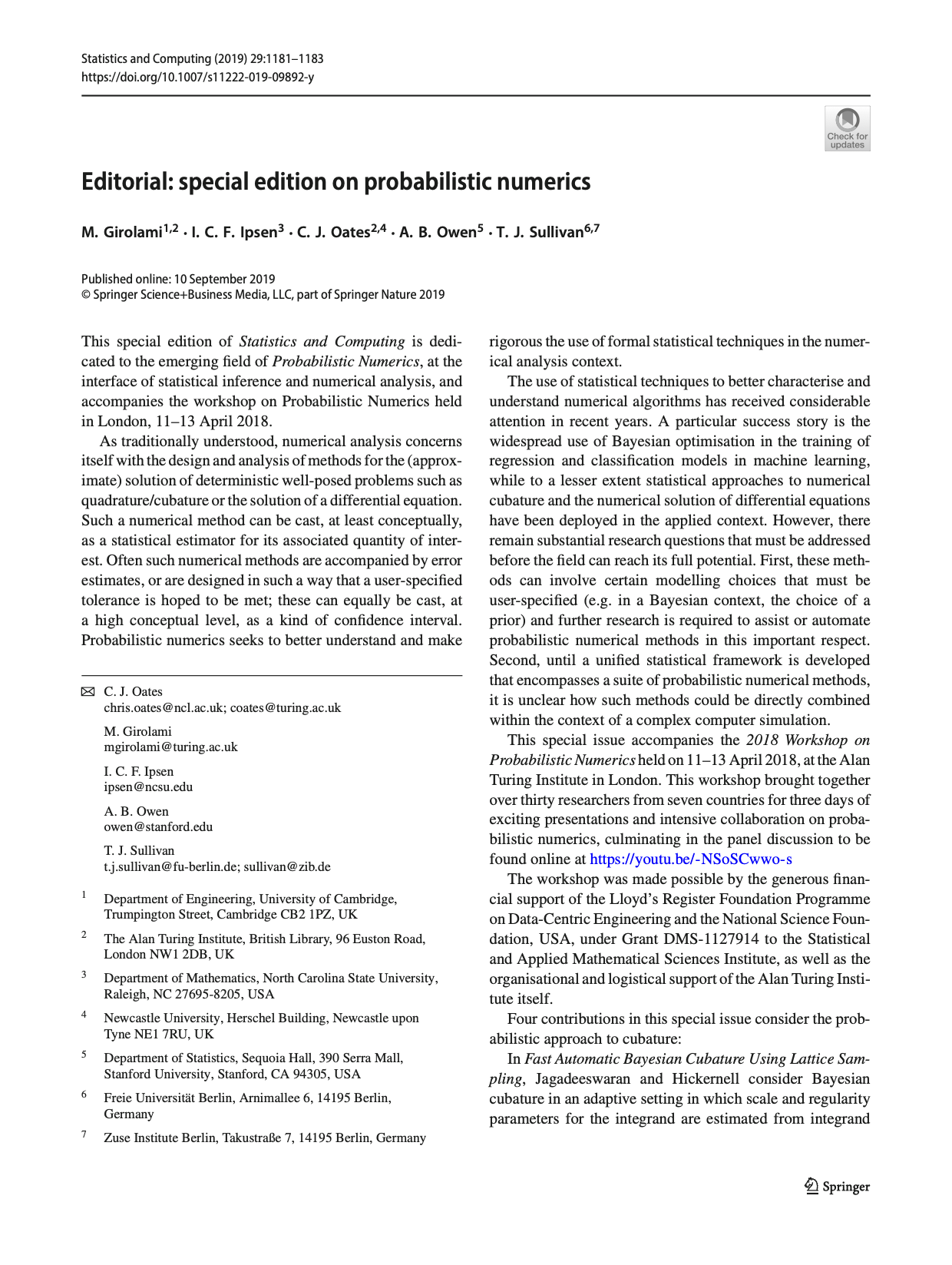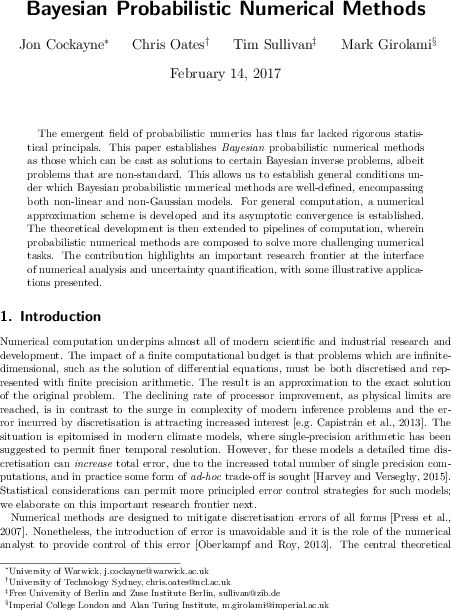# Tim Sullivan

### #girolami

Clear Search### Optimality of probabilistic numerical methods

The paper “Optimality criteria for probabilistic numerical methods” by Chris Oates, Jon Cockayne, Dennis Prangle, Mark Girolami, and myself has just appeared in print:

C. J. Oates, J. Cockayne, D. Prangle, T. J. Sullivan, and M. Girolami. “Optimality criteria for probabilistic numerical methods” in Multivariate Algorithms and Information-Based Complexity, ed. F. J. Hickernell and P. Kritzer. Radon Series on Computational and Applied Mathematics 27:65–88, 2020. doi:10.1515/9783110635461-005

Abstract. It is well understood that Bayesian decision theory and average case analysis are essentially identical. However, if one is interested in performing uncertainty quantification for a numerical task, it can be argued that standard approaches from the decision-theoretic framework are neither appropriate nor sufficient. Instead, we consider a particular optimality criterion from Bayesian experimental design and study its implied optimal information in the numerical context. This information is demonstrated to differ, in general, from the information that would be used in an average-case-optimal numerical method. The explicit connection to Bayesian experimental design suggests several distinct regimes, in which optimal probabilistic numerical methods can be developed.

Published on Sunday 31 May 2020 at 08:00 UTC #publication #prob-num #oates #cockayne #prangle #girolami### Bayesian probabilistic numerical methods in SIAM Review

The 2019 Q4 issue of SIAM Review will carry an article by Jon Cockayne, Chris Oates, Mark Girolami, and myself on the Bayesian formulation of probabilistic numerical methods, i.e. the interpretation of deterministic numerical tasks such as quadrature and the solution of ordinary and partial differential equations as (Bayesian) statistical inference tasks.

J. Cockayne, C. J. Oates, T. J. Sullivan, and M. Girolami. “Bayesian probabilistic numerical methods.” SIAM Review 61(4):756–789, 2019. doi:10.1137/17M1139357

Abstract. Over forty years ago average-case error was proposed in the applied mathematics literature as an alternative criterion with which to assess numerical methods. In contrast to worst-case error, this criterion relies on the construction of a probability measure over candidate numerical tasks, and numerical methods are assessed based on their average performance over those tasks with respect to the measure. This paper goes further and establishes Bayesian probabilistic numerical methods as solutions to certain inverse problems based upon the numerical task within the Bayesian framework. This allows us to establish general conditions under which Bayesian probabilistic numerical methods are well defined, encompassing both the nonlinear and non-Gaussian contexts. For general computation, a numerical approximation scheme is proposed and its asymptotic convergence established. The theoretical development is extended to pipelines of computation, wherein probabilistic numerical methods are composed to solve more challenging numerical tasks. The contribution highlights an important research frontier at the interface of numerical analysis and uncertainty quantification, and a challenging industrial application is presented.

Published on Thursday 7 November 2019 at 07:00 UTC #publication #bayesian #siam-review #prob-num #cockayne #girolami #oates### Statistics and Computing special issue on Probabilistic Numerics

It is a great pleasure to announce that a special issue of Statistics and Computing (vol. 29, no. 6) dedicated to the theme of probabilistic numerics is now fully available online in print. This special issue, edited by Mark Girolami, Ilse Ipsen, Chris Oates, Art Owen, and myself, accompanies the 2018 Workshop on Probabilistic Numerics held at the Alan Turing Institute in London.

The special issue consists of a short editorial and ten full-length peer-reviewed research articles:

1. “De-noising by thresholding operator adapted wavelets” by G. R. Yoo and H. Owhadi
2. “Optimal Monte Carlo integration on closed manifolds” by M. Ehler, M. Gräf, and C. J. Oates
3. “Fast automatic Bayesian cubature using lattice sampling” by R. Jagadeeswaran and F. J. Hickernell
4. “Symmetry exploits for Bayesian cubature methods” by T. Karvonen, S. Särkkä, and C. J. Oates
5. “Probabilistic linear solvers: a unifying view” by S. Bartels, J. Cockayne, I. C. F. Ipsen, and P. Hennig
6. “Strong convergence rates of probabilistic integrators for ordinary differential equations” by H. C. Lie, A. M. Stuart, and T. J. Sullivan
7. “Adaptive step-size selection for state-space based probabilistic differential equation solvers” by O. A. Chkrebtii and D. A. Campbell
8. “Probabilistic solutions to ordinary differential equations as non-linear Bayesian filtering: A new perspective” by F. Tronarp, H. Kersting, S. Särkkä, and P. Hennig
9. “On the positivity and magnitudes of Bayesian quadrature weights” by T. Karvonen, M. Kanagawa, and S. Särkkä
10. “A modern retrospective on probabilistic numerics” by C. J. Oates and T. J. Sullivan

Published on Wednesday 30 October 2019 at 12:00 UTC #stco #prob-num #girolami #ipsen #oates #owen### Optimality of probabilistic numerical methods

Chris Oates, Jon Cockayne, Dennis Prangle, Mark Girolami, and I have just uploaded a preprint of our paper “Optimality criteria for probabilistic numerical methods” to the arXiv.

Abstract. It is well understood that Bayesian decision theory and average case analysis are essentially identical. However, if one is interested in performing uncertainty quantification for a numerical task, it can be argued that the decision-theoretic framework is neither appropriate nor sufficient. To this end, we consider an alternative optimality criterion from Bayesian experimental design and study its implied optimal information in the numerical context. This information is demonstrated to differ, in general, from the information that would be used in an average-case-optimal numerical method. The explicit connection to Bayesian experimental design suggests several distinct regimes in which optimal probabilistic numerical methods can be developed.

Published on Tuesday 15 January 2019 at 11:00 UTC #preprint #prob-num #oates #cockayne #prangle #girolami### Bayesian probabilistic numerical methods

Jon Cockayne, Chris Oates, Mark Girolami and I have just uploaded a preprint of our latest paper, “Bayesian probabilistic numerical methods” to the arXiv. Following on from our earlier work “Probabilistic meshless methods for partial differential equations and Bayesian inverse problems”, our aim is to provide some rigorous theoretical underpinnings for the emerging field of probabilistic numerics, and in particular to define what it means for such a method to be “Bayesian”, by connecting with the established theories of Bayesian inversion and disintegration of measures.

Abstract. The emergent field of probabilistic numerics has thus far lacked rigorous statistical principals. This paper establishes Bayesian probabilistic numerical methods as those which can be cast as solutions to certain Bayesian inverse problems, albeit problems that are non-standard. This allows us to establish general conditions under which Bayesian probabilistic numerical methods are well-defined, encompassing both non-linear and non-Gaussian models. For general computation, a numerical approximation scheme is developed and its asymptotic convergence is established. The theoretical development is then extended to pipelines of computation, wherein probabilistic numerical methods are composed to solve more challenging numerical tasks. The contribution highlights an important research frontier at the interface of numerical analysis and uncertainty quantification, with some illustrative applications presented.

Published on Tuesday 14 February 2017 at 12:00 UTC #preprint #prob-num #bayesian #cockayne #oates #girolami